Fields and Games

loopspace

2nd June 2016

# 1 Introduction

There's a fun pattern matching game called Dobble. As with many good games, it's based on a straightforward idea. There are $55$ cards (which happen to be circular, which means that the cards don't have a fixed orientation). Each card has $8$ symbols on it, drawn from a collection of $50$ symbols overall. The symbols on the cards are chosen in such a way that each pair of cards has exactly one symbol in common. The game is basically to spot the matching symbol on a given pair of cards1.

1There are different wrappings around this gameplay to add a bit of variation, and there's an extra wriggle in that the symbols may appear at different sizes and in different orientations.

As well as enjoying playing it, I've been convinced that there's something mathematical in there. Not particularly in the game play itself, but somewhere in the cards. There ought to be some relationship between the number of cards, number of symbols, and number of symbols per card.

# 2 Finding the Formula

I'll admit, there were a couple of reasons why I didn't find a formula very quickly. One is that it doesn't use my area of expertise. Another is that I wasn't thinking about it very hard. Lastly, I got a bit caught up in edge cases.

The edge cases are important to deal with. One way to artificially inflate the complexity is to add unique symbols to each card. This increases the number of symbols and symbols per card but without increasing the mathematical complexity of the set-up2. The other edge case is when there is a single symbol, then there can be any number of cards with that single symbol on it. This would make for a rather dull game!

2It increases the complexity of the game because the extra symbols add distractions, but that's not what we're interested in here.

So let's call a set of cards and symbols reduced if all symbols appear in at least two cards and all cards are unique. In particular, this means that if we only have one symbol then we only have one card.

# 3 The Junior Game

One thing mathematicians like to do when studying a problem is to consider simpler cases of it. So let's simplify our game. Choosing the right simplification is an art in itself, a little experimentation lead me to decide on $4$ symbols per card3. So we start with a pack with $4$ symbols per card and assume that it is reduced.

3Three or two symbols per card is too simple, five introduces extra complications, and while six is again more straightforward it's getting close to the original of eight.

Pick a card, any card4. We'll call this the base card. We're assuming four symbols per card, and it's clearly not important what those symbols actually are, so we'll take these as $♣$, $♢$, $♡$, and $♠$.

4Sorry.

Each other card in the deck has exactly one symbol in common with the base card, so split the deck according to the symbols on the base card. This forms $4$ sets of cards (we're tacitly using the assumption that our pack is reduced here to deduce that the sets are not empty).

Let's look at one of these sets, say $♣$. Each card in this set has the same symbol, $♣$, in common with the base card and therefore with each other. All the other symbols on the cards in this set must therefore be unique amongst these cards. As we've accounted for one symbol on each card, this leaves $3$ symbols per card. Let's use letters for these symbols. We don't know how many cards there are, but the first few would look like the following, wherein each row is a card:

 $\begin{array}{cccc}♣& a& b& c\\ ♣& d& e& f\\ ♣& g& h& i\\ ♣& j& k& l\\ ♣& m& n& o\\ ♣& p& q& r\end{array}$

As we have at least two symbols per card, in particular on the base card, there is another pile of cards sharing one of the other symbols on the base card. Let's take $♢$.

Take a card from this second set. It must share a symbol in common with each card from our first pile. That can't be the $♣$ because then it would have two symbols in common with the base card ($♣$ and $♢$). So it must have one of the other symbols from each card in the first pile.

By looking at the first card in the $♣$ set, our new card must have one of $a$, $b$, or $c$ on it. Let's assume5 that it is $a$. By looking at the second card, our new card must have one of $d$, $e$, or $f$. Again, wlog, let's assume $d$. From the third card, we assume $g$. At this point our new card looks like $\left\{♢,a,d,g\right\}$. The crucial point is that this is four symbols and so our card is now full.

5There's a classic mathematical phrase in use here: "without loss of generality", or "wlog", meaning that this assumption does not restrict our argument in any way.

In particular, there's no room for a symbol in common with the fourth card in our first set, $\left\{♣,j,k,l\right\}$, and so that card cannot exist. Our first set, therefore, can have at most $3$ cards in it:

 $\begin{array}{cccc}♣& a& b& c\\ ♣& d& e& f\\ ♣& g& h& i\end{array}$

There was clearly nothing special about $♣$ here so this argument holds for each of the sets. Thus each set has at most $3$ cards in it and so the number of cards in the deck is at most $3×4+1=13$ (the $1$ is the base card). Note that the significance of the $3$ here is that it is the number of symbols on a card other than the symbol in common with the base card. Thus $3$ here is "$4-1$".

This is an upper bound on the number of cards. We haven't shown that there is a deck with that number of cards.

There's a "without loss of generality" argument that says that if the $♣$ cards are as above, then we can assume that the $♢$ cards are:

 $\begin{array}{cccc}♢& a& d& g\\ ♢& b& e& h\\ ♢& c& f& i\end{array}$

To put this another way, if we make a grid of the extra symbols:

 $\begin{array}{ccc}a& b& c\\ d& e& f\\ g& h& i\end{array}$

then the $♣$ cards are the rows and the $♢$ cards are the columns. For the $♡$ and $♠$ cards we need to find sets of three symbols from this grid with the property that each set has one from each row and one from each column. This will ensure a one-symbol overlap with each card from the $♣$ and $♢$ piles. In addition, the $♡$ sets must be disjoint from each other (as these already share the $♡$ symbol), and similarly for the $♠$ sets.

This is possible. For $♡$, we start with the downward diagonal, $\left\{a,e,i\right\}$. The disjoint property then forces us to take $\left\{b,f,g\right\}$ and $\left\{c,d,h\right\}$. For $♠$, we start with the upward diagonal, $\left\{g,e,c\right\}$, which forces $\left\{h,f,a\right\}$ and $\left\{i,d,b\right\}$.

We should check that this works, but we can do this by just "look and see". The full list of cards is then:

 $\begin{array}{cccc}♣& ♢& ♡& ♠\\ ♣& a& b& c\\ ♣& d& e& f\\ ♣& g& h& i\\ ♢& a& d& g\\ ♢& b& e& h\\ ♢& c& f& i\\ ♡& a& e& i\\ ♡& b& f& g\\ ♡& c& d& h\\ ♠& g& e& c\\ ♠& h& f& a\\ ♠& i& d& b\end{array}$

# 4 The General Story

Let's see what of the above generalises. We start with a pack with $k$ symbols per card. Exactly as above, we pick a base card and split the rest of the pack accordingly. The same argument runs through to show that there can be at most $k-1$ cards in each of the resulting piles. This gives at the maximum number of cards:

 $k\left(k-1\right)+1={k}^{2}-k+1$

Similarly, for symbols if one pile is maximal (has $k-1$ cards in it) then the number of symbols is:

 $\left(k-1{\right)}^{2}+k={k}^{2}-2k+1+k={k}^{2}-k+1$

The part that is trickier is to show that these upper bounds are achievable.

In the following, $k-1$ is going to play a bigger role than $k$ so we'll give that a symbol of its own. For not completely arbitrary reasons, we'll call it $p$. So $p=k-1$.

The clue comes from laying out the grids in a particular fashion. In our simpler example, let us copy the last two rows of the grid at the start:

 $\begin{array}{ccc}d& e& f\\ g& h& i\\ a& b& c\\ d& e& f\\ g& h& i\end{array}$

The $♡$ cards were $\left\{a,e,i\right\}$, $\left\{b,f,g\right\}$, and $\left\{c,d,h\right\}$. The first of these, $\left\{a,e,i\right\}$, we said was the downward diagonal but now we can see that the others are similar downward lines parallel to the first. Similarly, the $♠$ cards are the upward diagonal and the lines parallel to it.

Once the word "line" has been said, more ideas should follow. We can draw all of the cards as lines on the above grid, resulting in Figure 1.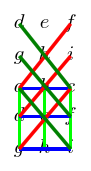Figure 1: The cards as lines.

Drawing lines is more than just a convenient illustration. Cards in the same pile should have empty intersection while cards in different piles should intersect in a single symbol. If we replace "card" with "line" and "symbol" with "point" this translates to: lines in the same pile should not intersect while lines in different piles should intersect in a single point. Or in more geometrical language, lines in the same pile should be parallel while lines in different piles should not be parallel.

Let's make this more precise. We place the symbols on the cartesian plane at the points with integer coordinates, starting at $\left(0,0\right)$. We then repeat the pattern vertically up the plane. So as we go up, every $p$th symbol is the same. We shall represent the symbols as dots, relying on their coordinates to tell us when two points represent the same symbol. The result is shown in Figure 2.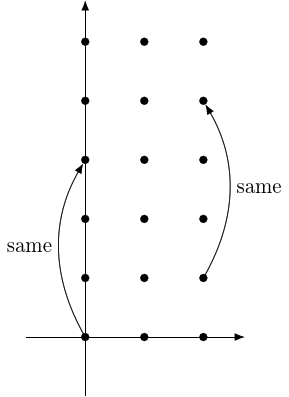Figure 2: The symbols on a grid.

Now we usually think of lines in terms of gradient and $y$–intercept, so we'll do the same here. Parallel lines have the same gradient, so the cards in the same pile must differ by $y$–intercept. Fortunately, we have $p$ unique possibilities for the $y$–intercepts, starting at $0$ and going up to $p-1$ (but no more, as the symbol at $\left(0,p\right)$ is the same as that at $\left(0,0\right)$).

Looking at the possible gradients, let's start with the lines through the origin. The gradient then says which symbol in the second column (with $y$–coordinate $1$) lies on this line. We could experiment with strange gradients, but again we have some obvious choices: the numbers $0$ to $p-1$.

So we have gradients $0$ to $p-1$ and $y$–intercepts $0$ to $p-1$. This doesn't quite give enough as we need one more set of lines, but for these we take the vertical lines. Figure 3 shows the resulting picture.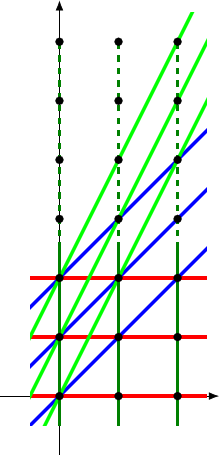Figure 3: The different lines.

Finally, to get the symbols on the cards we take the $p$ symbols that lie on the line. For all but the vertical lines, these are the symbols corresponding to the actual points they pass through. For the vertical lines, we have to remember that the symbols repeat and so once we have collected the first $p$ symbols we can stop.

This all looks good: our knowledge of lines says that the families of parallel lines don't intersect, and non-parallel lines intersect in a single point.

However, this ignores the fact that the symbols repeat and it is the symbols that count, not the points. In the example we've been using then actually all is fine, but if we increase to $p=4$ we run into problems. Figure 4 shows the problem.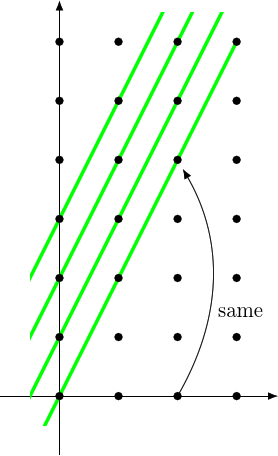Figure 4: The case $p=4$.

The green lines have gradient $2$, with the base green line being $y=2x$. This starts at $\left(0,0\right)$. It passes through the point $\left(2,4\right)$, which is the same symbol as $\left(2,0\right)$. This is a problem because the points $\left(0,0\right)$ and $\left(2,0\right)$ lie on the same horizontal line so the line $y=2x$ has two symbols in common with that horizontal line.

It turns out that the case $p=4$ can be fixed, but $p=6$ cannot. In general, this strategy works as stated when $p$ is prime. When $p$ is a prime power, such as $4$ or $8$ or $9$, then there is a variant of this strategy that works. But if $p$ has two distinct prime factors, this strategy will fail.

So $p=6$ is the smallest number where we don't have a proof that the maximum number of cards is achievable.

# 5 The Composite Case

Unfortunately, this isn't a proof that it can't be done for $p=6$ or $p=10$ or any of these numbers. It just says that the "obvious" method will fail. I don't know of a method to show that the case $p=6$ can't be done, as there may be some cunning way of stacking the deck6 to get the required symbols.

6Couldn't resist that one. Sorry.

But I doubt it. The reason that I doubt it is that I wrote a computer program to search for a solution and it hasn't found one.

Yet.

It hasn't finished looking though. There are a lot of cases to consider and I've probably not programmed it to look as carefully as possible so it takes a long time. However, the same program found a solution for $p=7$ in far less time than it's spent looking for solutions for $p=6$ so my hunch is that no solution exists for $p=6$.

# 6 The Wrap Around

Back to the simple cases that do work. We fix $p$ a prime number. The key is to work with modular arithmetic, which is sometimes called clock arithmetic. In short, you imagine that you are working with the numbers on a clock only instead of a traditional $12$hr clock the dial is marked with the numbers $0$ to $p-1$.

The key feature of modular arithmetic is that you "wrap around" the clock. So whenever a calculation takes you outside the region $0$ to $p-1$ you wrap around the clock to work out what the answer should be. For example, on a normal clock then $3$hrs past $10$ o' clock should be $13$ o' clock but due to the "wrap around" it is the same as $1$ o' clock7. This extends to subtraction, so $3$hrs before $1$ o' clock is $10$ o' clock. It also extends to multiplication: simply work out the multiplication as normal and then "wrap" the answer around the clock. So $2$ lots of $10$hrs is $20$hrs which on a $12$hr clock is $8$ o' clock.

7The key difference to a normal clock – apart from the number of "hours" – is that $12$ o' clock is written $0$.

On "most" clocks, this doesn't extend to division. But if the clock number is prime then division works. The key is to remember that division is "that which undoes multiplication". If you multiply by, say, $3$ and then divide by $3$ you should get back to where you started. On a $12$hr clock then that can't be done. But on, say, a $5$hr clock then it can. On a $5$hr clock then multiplying by $3$ and then by $2$ gets you back where you started, as in Table 1.

 $×3$ $×2$ $0$ $↦$ $0$ $↦$ $0$ $1$ $↦$ $3$ $↦$ $6\to 1$ $2$ $↦$ $6\to 1$ $↦$ $2$ $3$ $↦$ $9\to 4$ $↦$ $8\to 3$ $4$ $↦$ $12\to 2$ $↦$ $4$

Table 1: Multiplying by $3$ and then $2$ on a $5$hr clock.

This extends more generally: if, and only if, $p$ is prime then division works on a $p$hr clock.

How can we use this? Instead of repeating our grid on the normal axes, we label the points using the numbers on a $p$hr clock. When we work out lines, we use clock arithmetic. So with our example of $p=3$, the line $y=2x$ passes through the points $\left(0,0\right)$, $\left(1,2\right)$, and $\left(2,1\right)$. The latter because when $x=2$ then $y=2×2=4$ but on the $3$hr clock then $4$ is the same as $1$.

Joining the points together becomes a little dubious, as there are no fractions or decimals in clock arithmetic, but it's convenient to help see which points are connected. It's worth looking at Figure 5 to see that the "lines" are as expected. The red lines are $y=0x+c$, the blue lines are $y=x+c$, the green lines are $y=2x+c$, and the dark green lines are the vertical lines.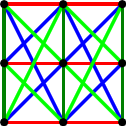Figure 5: The lines on a clock.

Our general strategy is the same as before: we define lines by $y=mx+c$ with $0\le m,c\le p-1$. What we need to show is that the key properties of lines carry over from normal space into this strange clock space. That is, parallel lines don't intersect and non-parallel lines intersect in a single point.

That parallel lines don't intersect is straightforward. Lines are parallel if they have the same gradient so we're asking for the intersection of $y=mx+c$ and $y=mx+d$. This occurs if $mx+c=mx+d$. The rules of algebra still apply so we can simplify this to $c=d$. Thus parallel lines intersect only if they are the same line.

Now let us look at non-parallel lines, say $y=mx+c$ and $y=nx+d$. Then we solve:

 $\begin{array}{rl}mx+c& =nx+d\\ mx-nx& =d-c\\ \left(m-n\right)x& =d-c\end{array}$

Now we use division and "divide" by $m-n$ to get:

 $x=\frac{d-c}{m-n}$

where the fraction has to be interpreted in clock arithmetic as meaning $\left(d-c\right)$ divided by $\left(m-n\right)$.

This shows that $x$ is unique on the clock and so there is a single intersection point.

Thus our intuition works and non-parallel lines intersect at a single point. This means that the strategy for making a deck works when $p$ is prime.

There is an adaptation of this for when $p$ is a prime power, meaning a prime number raised to some power. There's a "clock" for these situations, but it isn't the clock with numbers $0,1,\dots ,p-1$. For example, the clock for $4$ has four symbols $\left\{0,1,\alpha ,1+\alpha \right\}$ and addition works like this:

 $\begin{array}{rl}0+0& =0\\ 0+1& =1\\ 0+\alpha & =\alpha \\ 0+\left(1+\alpha \right)& =1+\alpha \\ 1+1& =0\\ 1+\alpha & =1+\alpha \\ 1+\left(1+\alpha \right)& =\alpha \\ \alpha +\alpha & =0\\ \alpha +\left(1+\alpha \right)& =1\\ \left(1+\alpha \right)+\left(1+\alpha \right)& =0\end{array}$

Multiplication works like this:

 $\begin{array}{rl}0×0& =0\\ 0×1& =0\\ 0×\alpha & =0\\ 0×\left(1+\alpha \right)& =0\\ 1×1& =1\\ 1×\alpha & =\alpha \\ 1×\left(1+\alpha \right)& =1+\alpha \\ \alpha ×\alpha & =1+\alpha \\ \alpha ×\left(1+\alpha \right)& =1\\ \left(1+\alpha \right)×\left(1+\alpha \right)& =\alpha \end{array}$

# 7 To Infinity and Beyond

We're not done. We're going to extend our clocks a little more. If we take seriously the analogy between "cards" and "lines" then our geometrical picture doesn't reflect the whole story. The reason we wanted some lines to be parallel was because we had already dealt with their intersections. But in the full deck, every pair of cards intersects. So to put the full deck into a geometrical picture, we need for all lines to intersect somewhere, whether parallel or not.

The oft-heard maxim is that parallel lines meet "at infinity". As with so many such sayings, it is completely false: there is no "infinity" in ordinary geometry for parallel lines to meet at. But also as with so many such sayings, mathematicians have taken this saying and made it work.

To make parallel lines meet "at infinity" we need to add in a point "at infinity" for them to meet at. But we have to do this carefully. We can't just add one point "at infinity" and have all lines meet there because then the non-parallel lines will meet twice: once in "normal space" and once "at infinity". So we need to add a point "at infinity" for each possible gradient, including the vertical lines (whose gradient could well be said to be "infinity").

Thus the grid for $p=3$ should look like Figure 6.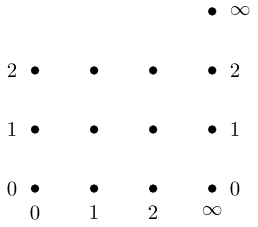Figure 6: Points at infinity.

With the points at infinity added, the line $y=mx+c$ then passes through the point $\left(\infty ,m\right)$ and vertical lines through $\left(\infty ,\infty \right)$. In addition, we get a further vertical line passing through all the points "at infinity". Our final diagram is in Figure 7.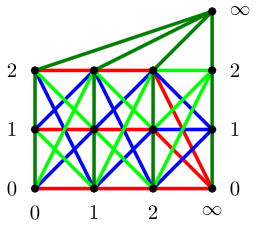Figure 7: Points at infinity.

With regard to counting, we now have ${p}^{2}+p+1$ symbols, but also ${p}^{2}+p+1$ lines (${p}^{2}$ lines of the form $y=mx+c$, $p$ "normal" vertical lines, and $1$ vertical line "at infinity"). This is no coincidence. The lines are specified by a $y$–intercept and a gradient, which means $2$ numbers, and the symbols are specified by an $x$–coordinate and a $y$–coordinate, which is again $2$ numbers. The points "at infinity" fit into this story as the vertical lines. So there is a duality between points and lines, which transfers to a duality between cards and symbols.

Moreover, there are $p+1$ points on each line and thus $p+1$ points on each card. The number of cards and symbols agrees with our earlier calculation since if $p=k-1$ then

 ${p}^{2}+p+1=\left(k-1{\right)}^{2}+\left(k-1\right)+1={k}^{2}-2k+1+k-1+1={k}^{2}-k+1$

# 8 Back in the Game

The Dobble pack has $8$ symbols per card, which fits our pattern as $8-1=7$ is prime. However, there are only $55$ cards in the Dobble pack whereas ${8}^{2}-8+1=57$. So if Dobble have used the correct scheme, there are two cards missing8. Rather than figure out if Dobble used the right scheme, the easiest way is to find candidates for the missing cards and observe that they work.

8It is unlikely, but possible, that there is a way to choose the symbols on the cards so that although the pack is not its maximum size then it isn't possible to simply add two cards to make it the maximum size.

Using the system of dividing the pack into piles according to a "base" card, it is possible to find the two missing cards. These are9:

9Your family might have different names for the symbols

• Ice cube, cactus, T-rex, question mark, snowman, maple leaf, daisy, man.

• Skull, bulb, dog, hammer, snowman, exclamation mark, eye, ladybird.

There are, though, $57$ symbols10.

10On the game instructions, they say "$50$ symbols".

There's probably something optimal in terms of game play in having $8$ symbols per card. If you want a simpler or slightly more difficult game, Table 2 gives the number of maximum number of cards for a given number of symbols per card. Note that $k-1$ has to be a prime power, so $7=6+1$ and $11=10+1$ are omitted from the table.

 Symbols per card Pack size $2$ $3$ $3$ $7$ $4$ $13$ $5$ $21$ $6$ $31$ $8$ $57$ $9$ $73$ $10$ $91$ $12$ $133$

Table 2: Pack size for different numbers of symbols per card.

# 9 And Finally …

The story is fairly complete if the number of symbols on a card is one more than a prime power and you want the maximum number of cards. But there are still some questions that could be investigated.

1. Is it possible to find a configuration of symbols and cards with more symbols than the "maximum"?

The point here is that our "maximum" number of symbols was predicated on a certain assumption about the number of cards. If that assumption is false, is it possible to get more symbols?

2. What's the largest deck with $7$ symbols per card?

The largest my computer search found was $23$, but that wasn't a full search.

3. For a given number of symbols per card, are there maximal configurations of cards that are not the maximum?

That is, if we have, for example, $8$ symbols per card then the maximum number of cards is $57$. Clearly we can get a smaller deck by simply discarding cards, but there will almost certainly be other configurations with fewer than $57$ cards with the property that it is impossible to add cards to them.